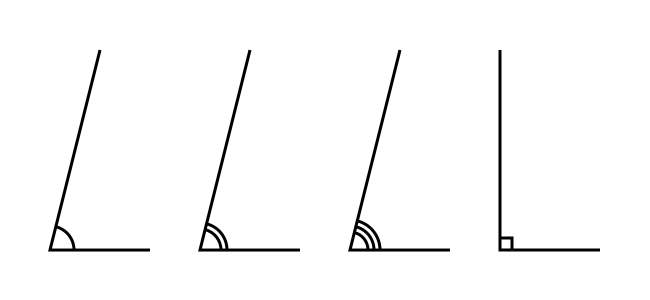# Angle marker

Martin McBride, 2020-08-23
Tags geometry angle
Categories generativepy generative art

The angle_marker function adds an angle marker to the path, that can then be stroked. Angle markers look like this:An angle marker can have 1, 2 or 3 arcs. The 2 and 3 arc forms are often used to indicate that two angles are equal. You can also draw a right angle marker, as shown.

## angle_marker function

angle_marker(ctx, a, b, c, count=1, radius=8, gap=2, right_angle=False)

Parameter Type Description
ctx Context The Pycairo Context to draw to
a (number, number) Tuple (x, y) for point a
b (number, number) Tuple (x, y) for point b
c (number, number) Tuple (x, y) for point c
count int Number of arcs on angle, 1, 2 or 3.
gap number Gap between arcs in user units.
right_angle boolean True for a right angle.

Draws an angle arc for the angle abc. The arc is centred on point b, and shows the angle between lines ab and cb in the clockwise direction.

If you are using a flipped coordinate system (see the setup function in the drawing module), the angle is drawn in the counterclockwise direction.

count allows you to draw double or triple arcs to indicate different pairs of equal angles. If count is not 1, gap sets the distance between the arcs - typically a value that is about twice the line thickness usually looks best.

right_angle can be set to true to draw a right angle marker rather than an arc. In that case, count is ignored.

Note that it is up to your code do ensure that the angle actually is a right angle before setting this. If you set this flag when the angle isn't a right angle (or very close), you will get strange looking result. If you don't set this flag when the angle is a right angle, a normal angle arc will be drawn. This is to avoid spurious effects in animations if a changing angle happens to be exactly 90 degrees in one frame/

## Example

Here is an example that shows the angle_marker, tick and paratick functions in use:

from generativepy.drawing import make_image, setup
from generativepy.color import Color
from generativepy.geometry import line, polygon, angle_marker, tick, paratick

'''
Create rectangles using the geometry module.
'''

def draw(ctx, width, height, frame_no, frame_count):
setup(ctx, width, height, background=Color(0.8))

ctx.set_source_rgba(*Color(0, 0, 0.5))
ctx.set_line_width(3)

## Draw lines with ticks and paraticks
a = (50, 50)
b = (50, 150)
line(ctx, a, b)
ctx.stroke()
tick(ctx, a, b, length=12, gap=6)
ctx.stroke()

a = (100, 50)
b = (150, 150)
line(ctx, a, b)
ctx.stroke()
tick(ctx, a, b, 2, length=12, gap=6)
ctx.stroke()

a = (250, 50)
b = (200, 150)
line(ctx, a, b)
ctx.stroke()
tick(ctx, a, b, 3, length=12, gap=6)
ctx.stroke()

a = (350, 50)
b = (350, 150)
line(ctx, a, b)
ctx.stroke()
paratick(ctx, a, b, length=12, gap=6)
ctx.stroke()

a = (400, 50)
b = (450, 150)
line(ctx, a, b)
ctx.stroke()
paratick(ctx, a, b, 2, length=12, gap=6)
ctx.stroke()

a = (550, 150)
b = (500, 50)
line(ctx, a, b)
ctx.stroke()
paratick(ctx, a, b, 3, length=12, gap=6)
ctx.stroke()

## Draw lines with angles
a = (50, 250)
b = (50, 450)
c = (150, 450)
polygon(ctx, (a, b, c), closed=False)
ctx.stroke()
angle_marker(ctx, a, b, c, radius=24, gap=6, right_angle=True)
ctx.stroke()

a = (250, 250)
b = (200, 450)
c = (300, 450)
polygon(ctx, (a, b, c), closed=False)
ctx.stroke()
angle_marker(ctx, a, b, c, 3, radius=24, gap=6)
ctx.stroke()

a = (300, 250)
b = (400, 300)
c = (500, 300)
polygon(ctx, (a, b, c), closed=False)
ctx.stroke()
angle_marker(ctx, c, b, a, radius=24, gap=6)
ctx.stroke()

a = (300, 350)
b = (400, 400)
c = (500, 400)
polygon(ctx, (a, b, c), closed=False)
ctx.stroke()
angle_marker(ctx, a, b, c, 2, radius=24, gap=6)
ctx.stroke()

make_image("/tmp/geometry-markers.png", draw, 600, 500)